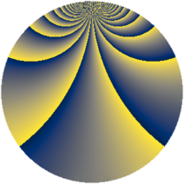# Properties

 Label 1610.2.beLevel $1610$ Weight $2$ Character orbit 1610.be Rep. character $\chi_{1610}(111,\cdot)$ Character field $\Q(\zeta_{22})$ Dimension $640$ Sturm bound $576$

# Related objects

## Defining parameters

 Level: $$N$$ $$=$$ $$1610 = 2 \cdot 5 \cdot 7 \cdot 23$$ Weight: $$k$$ $$=$$ $$2$$ Character orbit: $$[\chi]$$ $$=$$ 1610.be (of order $$22$$ and degree $$10$$) Character conductor: $$\operatorname{cond}(\chi)$$ $$=$$ $$161$$ Character field: $$\Q(\zeta_{22})$$ Sturm bound: $$576$$

## Dimensions

The following table gives the dimensions of various subspaces of $$M_{2}(1610, [\chi])$$.

Total New Old
Modular forms 2960 640 2320
Cusp forms 2800 640 2160
Eisenstein series 160 0 160

## Trace form

 $$640q - 64q^{4} + 72q^{9} + O(q^{10})$$ $$640q - 64q^{4} + 72q^{9} - 64q^{16} - 32q^{18} + 132q^{21} + 24q^{23} - 64q^{25} - 16q^{29} - 14q^{35} + 72q^{36} + 48q^{39} + 88q^{43} - 78q^{49} + 88q^{51} - 22q^{56} + 160q^{58} - 220q^{63} - 64q^{64} - 12q^{70} + 24q^{71} + 144q^{72} - 196q^{77} - 16q^{78} + 176q^{79} - 16q^{81} - 22q^{84} + 8q^{85} + 44q^{86} + 24q^{92} + 16q^{93} - 32q^{95} + 264q^{99} + O(q^{100})$$

## Decomposition of $$S_{2}^{\mathrm{new}}(1610, [\chi])$$ into newform subspaces

The newforms in this space have not yet been added to the LMFDB.

## Decomposition of $$S_{2}^{\mathrm{old}}(1610, [\chi])$$ into lower level spaces

$$S_{2}^{\mathrm{old}}(1610, [\chi]) \cong$$ $$S_{2}^{\mathrm{new}}(161, [\chi])$$$$^{\oplus 4}$$$$\oplus$$$$S_{2}^{\mathrm{new}}(322, [\chi])$$$$^{\oplus 2}$$$$\oplus$$$$S_{2}^{\mathrm{new}}(805, [\chi])$$$$^{\oplus 2}$$